Martin boundary in potential theory

The ideal boundary of a Green space(see also Boundary (in the theory of uniform algebras)), which allows one to construct the characteristic representation of positive harmonic functions in. Letbe a locally compact, non-compact, topological space, and letbe a family of continuous functions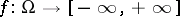. The Constantinescu–Cornea theorem  asserts that, up to a homeomorphism, there is a unique compact space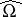with the following properties: 1)is an everywhere-dense subspace of; 2) each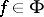extends continuously to a function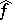on, separating points on the ideal boundary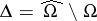ofrelative to; and 3)is an open set in.

Now, letbe a bounded domain in a Euclidean space,, or, more generally, a Green space; let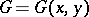be the Green function onwith poleand letbe fixed. The Martin space or Martin compactificationofis obtained via the Constantinescu–Cornea theorem by taking forthe family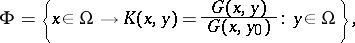where, by definition,. The Martin boundary is the corresponding ideal boundary. The Martin topologyis the topology on the Martin space. Two Martin spaces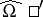,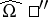corresponding to different points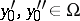are homeomorphic. The function, the extension of, is harmonic inand jointly continuous in the variables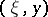;is a metrizable space. Martin's fundamental theorem  asserts: The class of all positive harmonic functionsonis characterized by the Martin representation:(*)

whereis a positive Radon measure on. The measurein (*) is not uniquely determined by the function. A harmonic function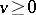is called minimal inif each harmonic functionsuch thatinis proportional to. Minimal harmonic functions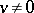are proportional to, the corresponding points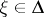are called minimal, and the set of minimal points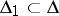is called the minimal Martin boundary. If one poses the additional condition thatin (*) be concentrated on, one obtains the canonical Martin representation:in which the measure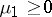is uniquely determined by.

Examples. a) If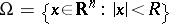is a ball of radiusin,, then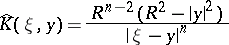is the Poisson kernel,is the Euclidean closure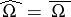, the Martin boundaryis the sphere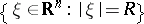, all points of which are minimal. The representation (*) in this case reduces to the Poisson–Herglotz formula (see Integral representation of an analytic function; Poisson integral).

b) The Martin boundarycoincides with the Euclidean boundary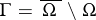wheneveris a sufficiently smooth hypersurface in,.

c) Ifis a simply-connected domain in the plane, then the Martin boundarycoincides with the set of limit elements, or Carathéodory prime ends. Thus, an element of the Martin boundarycan be considered as a generalization of the notion of a prime end to dimension.